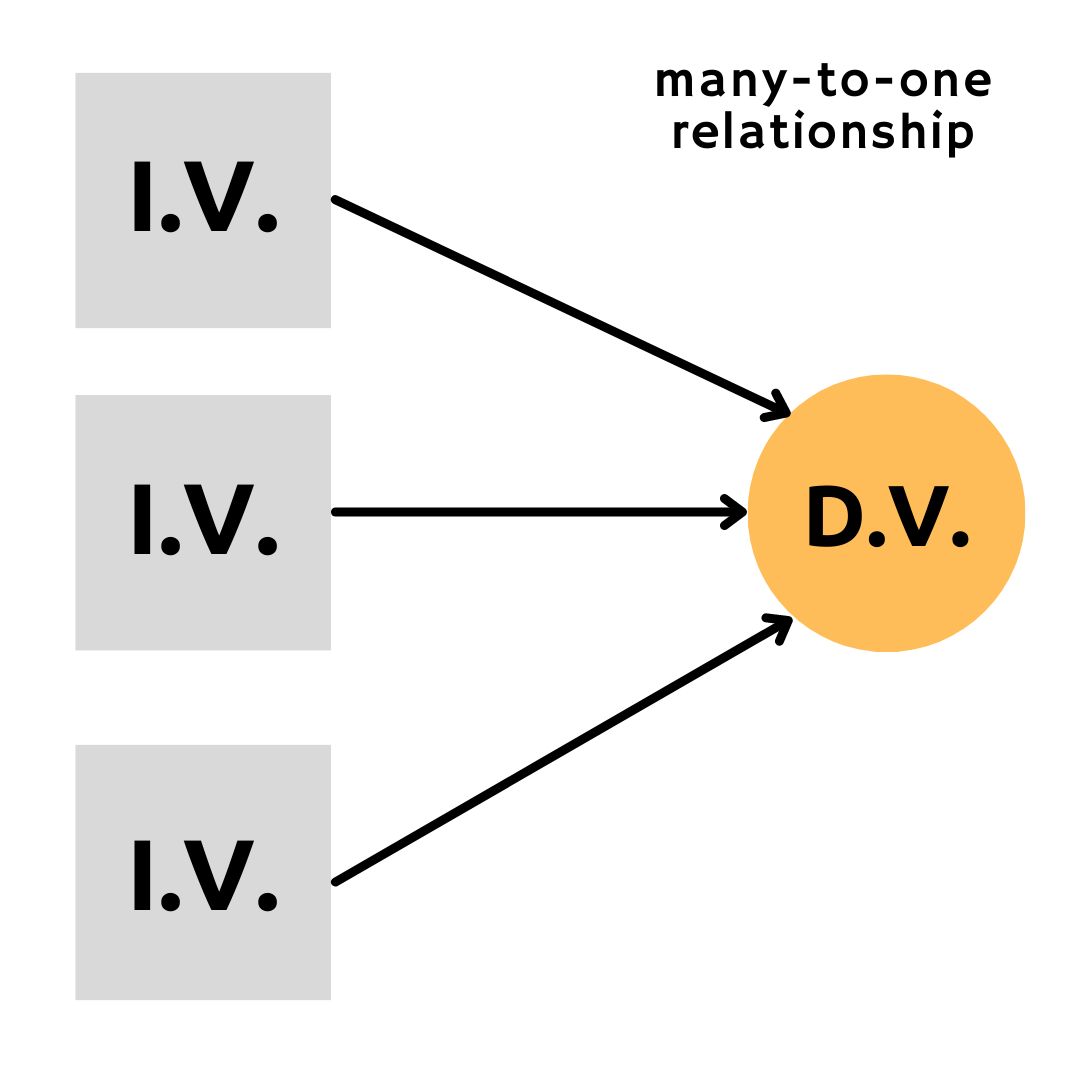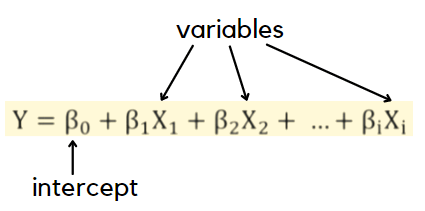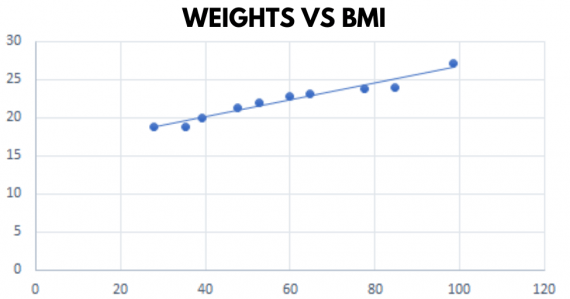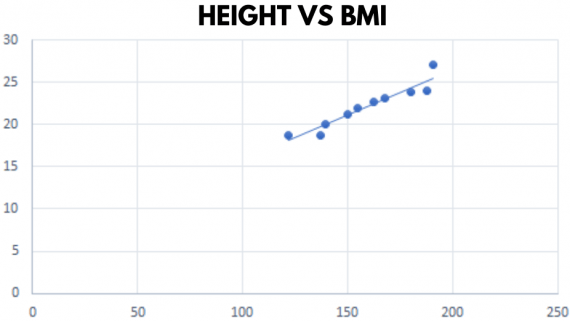Till now we studied the relationship between one dependent variable and one independent variable. What about the scenario when two or more variables?

Multiple Linear Regression is used to estimate the relationship between two or more independent variables and one dependent variable. Multiple linear regression acts as a statistical technique used to predict the outcome of a variable based on the value of two or more variables. It is also known simply as multiple regression.

Think it this way, your height depends on the nutrition you take. But is it the only factor? The height of your parents, your physical fitness, and your environment also play an important role. Thus, your height is dependent on more than one factor/variable.

Note: We will reserve the term multiple regression for models with two or more predictors(independent values) and one response(dependent values). There are regression models with two or more response variables which is not the case here.

In this chapter, we will learn a new method for computing the parameter estimates of multiple regression models. This method is more compact but convenient enough for cases when the number of unknown parameters is large. So let’s begin!

Relation with Linear Regression:

Remember that Linear Regression has one to one relationship. We utilized only one independent variable to explain the variation in the dependent variable. Now in multiple regression, we have many to one relationship. This implies that we have two or more independent variables to utilize to predict the variation in the value of a dependent variable.

Thus, Multiple Regression can also be referred to as an extension of linear regression.The addition of more independent variables creates more relationships among them. So not only are the independent variables potentially related to the dependent variable, but they are also related to each other. When this happens, it is called multicollinearity. For instance, consider you are eating your dinner and you put rock salt and table salt in your meal, all you know is that your meal tastes salty. But can you tell the difference between the two salts? No! This is because both the salts have now the same relationship with your dinner. There is no distinction left between the independent variables which create a problem to estimate which salt is more responsible for the saltiness in your meal.

Hence, it is ideal for all independent variables to be related to the dependent variable and not with each other.

Multiple Regression Equation

Earlier we studied the equation of line while deriving the equation for the Simple Linear Regression model. Here, it varies by changes in the number of variables. Let’s have a look.On the right-hand side of the equation, we have the sum of linear parameters. So, beta sub-zero which is our intercept then we have beta-one x-one which is the first variable and its coefficient, beta-two x-two which is the second variable along with its coefficient, and so on till ‘i’, that is the number of parameters considered.

Note: We are not considering the error term as of now.

So, we follow the same basic form! Intercept plus the coefficients paired with variable give the estimate for our multiple regression model.

Let’s start with an example for conducting our analysis of multiple regression. Consider a random sample where the height and weight of an individual are independent variables and BMI (Body Mass Index) acts as the dependent variable.

Weight (in kgs)
Height (in cms)
BMI
28.0
121.92
18.8
35.2
137.16
18.7
39.1
139.7
20.0
47.6
149.86
21.2
52.6
154.94
21.9
59.9
162.56
22.7
64.8
167.64
23.1
77.5
180.3
23.8
84.8
187.96
24.0
98.5
190.5
27.1

From the table, we know that an individual weighing 28kgs and have a height of 121.92 centimeters, owns a BMI of 18.8. BMI acts as the dependent variable i.e. y. Weight and Height are the independent variables denoted as x1, x2 respectively.

According to what we discussed earlier, there are 2 relationships to analyze with respect to the independent and dependent variable and 1 relationship between the independent variables. Thus, in total, we have 3 relationships to analyze. For checking multicollinearity in these 3 relationships.

1. Look at the independent variables to the dependent variable scatterplots.Recall forming regression equation of the above graph with the given data points. On doing that you may find the following equation for Weights(x1) and BMI(y).$y=0.1109*x1+15.608$

This means, increase in 1 kg of weight will increase BMI by 0.1109.Similarly, form a regression equation of the above graph with the given data points. On doing that you may find the following equation for Heights(x2) and BMI(y).$y=0.1077*x2+4.982$

This means, increase in 1 cm of height will increase BMI by 0.1077.

Summary: In the first case, our first independent variable, the weight of the individual has a strong linear relationship with BMI. Our second independent variable, the height of the individual also has a strong linear relationship with BMI as shown in the graph. Thus, BMI appears highly correlated with weight and height variables.

2. Look at the relation between the independent variables scatterplots.Now the above graph has a straight line that goes from the bottom left to the top right and shows us that we have a problem because the weight and height seem to be highly correlated, have a highly linear relationship. This means the model doesn’t know what coefficients to assign to these two variables if they seem so similar. Our model seems fine but this factor tells us we might have some problems. We will see that in further sections.

By now we have done some visual examination of lines from scatter plots. Let’s summarise!

Steps done:

1. Generate a list of potential variables, both independent and dependent.
2. Collect data according to the variables.
3. Check the relationship between each independent and dependent variable using scatterplots and correlations.
4. Check for multicollinearity among the independent variables.
5. Conduct simple linear regressions for each pair.
6. Use the non-redundant independent variables in the analysis to find the best-fitting model.
7. Use the best-fitting model for predicting the value of the dependent variable.

VIF (Variance Inflation Factor): It points out variables that are collinear that is, it points out multicollinearity. It finds how much the variance of an estimated regression coefficient increases if your predictors are correlated. Ranges for VIF:

If VIF is 1 then the model has no correlated factors. If VIF is between 5 and 10, it indicates a high correlation that may be problematic in some cases. If VIF goes above 10, you can tell the multicollinearity is present in the model.

Dummy Variable:

A dummy variable is an indicator variable to represent categorical data. It is also termed as quantitative variables.

It is considered that categorical data has two characteristics i.e. their range of values is restricted and hence they can take on only two quantitative values. Practically, regression results are easiest to predict when dummy variables are limited to two specific values, 1 or 0. Where, 1 represents the presence of a qualitative attribute, and 0 represents the absence.

For instance, consider an independent variable of a dataset as the type of vehicle with 3 types: SUV, Motorbike, or Bus. Now for building a regression model there are more than two characteristics and thus dummy variables are needed. For each type of vehicle, there are two characteristics as 0 and 1. Thus, each variable tells us if the vehicle is an SUV or it is a Motorbike or it is a bus.

Note: The number of dummy variables formed is one less than the total number of characteristics.

This means that if we consider the 2 dummy variables in the above example, we don’t need to know the values of the third variable. If the vehicle is an SUV, we know it is not a Motorbike or bus. If the vehicle is neither a bus nor an SUV, we know it is a Motorbike.

Implementation using S2/Kotlin

After all the understanding of concepts, it’s the coding time! So open your S2/Kotlin IDE and start by uploading your dataset. While constructing a regression equation, don’t forget to consider all your independent variables.

Model Training:

				
%use s2
//Entering the dataset
val sg: DataFrame = dataFrameOf(
"Weight", "Height", "BMI")(
28.0, 121.92, 18.8,
35.2, 137.16, 18.7,
39.1, 139.7, 20.0,
47.6, 149.86, 21.2,
52.6, 154.94, 21.9,
59.9, 162.56, 22.7,
64.8, 167.64, 23.1,
77.5, 180.3, 23.8,
84.8, 187.96, 24.0,
98.5, 190.5, 27.1,
)
println(sg)
//Constructing a Linear Problem Model
val problem = LMProblem(
DenseVector(sg["BMI"].asDoubles()),
//Considering all the independent variables
DenseMatrix(arrayOf(DenseVector(sg["Height"].asDoubles()).toArray(),DenseVector(sg["Weight"].asDoubles()).toArray())).t(),
true)
//run OLS regression
val ols = OLSRegression(problem)
// the regression coefficients
val beta_hat = ols.beta().betaHat()
// // the error terms
val residuals = ols.residuals()
println("beta_0 = ${beta_hat}, beta_1 =${beta_hat}")
//Beta is the slope value
println("R^2 = ${residuals.R2()}") //Residual is the difference between original and model value   Output:A DataFrame: 10 x 3 Weight Height BMI 1 28 121.92 18.8 2 35.2 137.16 18.7 3 39.1 139.7 20 4 47.6 149.86 21.2 5 52.6 154.94 21.9 6 59.9 162.56 22.7 7 64.8 167.64 23.1 8 77.5 180.3 23.8 9 84.8 187.96 24 10 98.5 190.5 27.1 beta_0 = -0.04892578154383942, beta_1 = 0.15907276557348418 R^2 = 0.9597966234364768 Model Testing: To code for this part, one should first know that this is called testing our model against the dataset we supplied to our model for training. So here we are gonna predict the value of BMI for a given value of Height and Weight. Add the following line of code in the end of the program written till now.   println("Expected value of BMI when Height = 150.0 and Weight = 53.4 is${ols.Ey(DenseVector(150.0,53.4))}")



Note: Adding more independent variables does not necessarily mean that the model will give better predictions; in fact, it can make things worse. This is called overfitting.

Let’s know more about this, consider that your multiple regression model explains 65% of variation in the dependent variable. In order to search for a better result, you start adding in more independent variables and variation improves. But it can do so under false pretenses. So adding more variables will explain more variation but it can cause other problems which we don’t want in our model. Thus, the idea is to pick the best variables instead of picking more variables for the model.

You are now aware about the structural concepts of predicting values with multiple independent variables.

We now conclude the topic, “Multiple Linear Regression”.

Hope you learned and practised new things in the world of prediction. Thank you for spending time on this course!